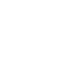# Widget Calculator

## The widget allows you to automatically calculate the data in the fields according to a given formula and display the result in the selected field.

Features and widget settings

The widget allows you to automatically calculate the data in the fields according to a given formula and display the result in the selected fieldThen you need to open the installed widget and click the “activate” buttonInstructions for the Calculator widget

To configure the widget, after installation, you need to go to "Settings".
Initially, in the "Settings" section there will be only the "Add Formula" button. After adding, a window will open with 3 tabs: "Formula", "Execution condition" and "Reference". Shown in the screenshot below.
1) In a tab "Formula" you will need to specify:• The name of the formula;
• A formula that depends on what needs to be calculated, for example, a percentage of a certain amount (It is worth noting that if you enter a percentage as a sign, the widget does not recognize this, percentages are calculated through a formula). +-/ works. Here we use @ to select fields from which numeric values will be taken.!! Important !!* The fields must be strictly numeric, otherwise, with complex formulas, the widget will fail. Also, you can add values to the formula from the "Reference" tab (information below), this is done through #.• Select rounding from the drop-down list;
• Select a numeric field in which the result of the calculation of the formula will be displayed;
• Indicate the figure, which formula, in which order will be considered.
2) In tab "Execution Conditions" you can specify for which inputs a certain formula should work.The fields and / or their value are indicated on the left and right, or your own numeral is entered in the right list (that is, if we select the responsible field in the left list, then in the right list it will give users who are in the system and further by analogy). The trigger condition is selected in the middle.
3) In tab "Directory" fields are selected, which we can later add to the formula through #. The field you add must be of type List or it won't even show up for selection.On the left, a "List" type field is selected. On the right, it fits what each position in the list will equal. After adding a directory, it can be substituted into the formula through #, and when a certain position is selected from the list in the lead card, the formula will calculate taking into account what the given position in the directory was equal to.
The calculation works without going to any stage, just click on the working field.
Need development for Kommo?
If you need functionality that is not in ready-made solutions, we can develop it